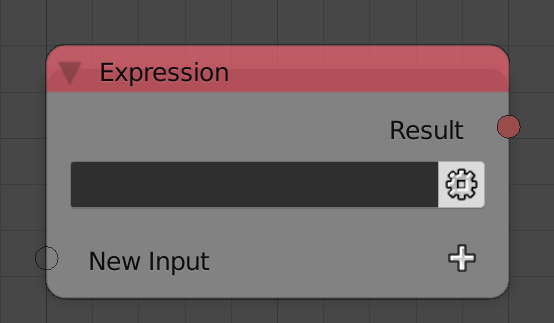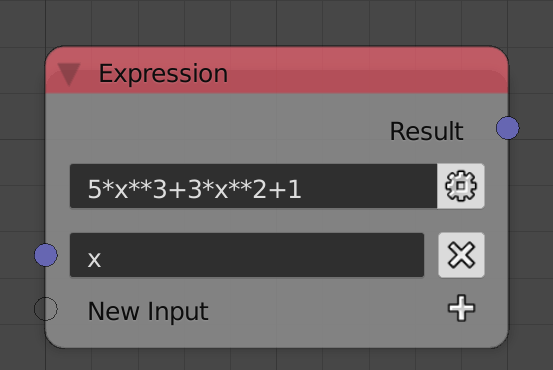# Expression

The expression node allows executing a single line of python code and return its value. The node can have multiple typed inputs which can be used as variables in the python expression. The inputs can’t be defined in the node itself because the space is reserved to the variable name representing the input.One of the simplest uses of this node is computing long mathematical equations. For instance, if one wants to compute the value of the polynomial `5x^3+3x^2+1` at some `x` one would have to add six nodes for multiplication, power and addition. Alternatively, one could just write that in an expression node as follows.• Modules - Importing python modules is done by writing their names with comma as a separator. For instance, if one wants to use `numpy` and `math` modules, one would write `numpy, math`.
• Debug Mode - If enabled, any errors will be caught and displayed in the node. If disabled, any error in the expression will cause a fatal error stopping the execution of the node tree. It should be noted that enabling debug mode slows down execution.
• Correct Type - If enabled, the node will attempt to convert the output to the required output type. If disabled, if the type output is different from the output type, the node will cause a fatal error stopping the execution of the node tree.
• Inline Expression - By default, modules are star imported and their attributes can be used directly. If this option is enabled, modules are normally imported and their attributes have to be preceded by the module name. For instance, in order to use the `argpartition` function from numpy one would write `numpy.argpartition` instead of `argpartition`. Enabling this option improves performance by avoiding start importing.
• Fixed Data Type - If disabled, the node will automatically change the type of the output socket based on what it gets connected to. If enabled, the type is fixed and doesn’t automatically change, in which case, the type can be defined using the gear button in the node.

## Python Inline Expressions

One is limited to a single line of code when using the expression node. Luckily, Python provides some features like conditional operators and list comprehensions that can provide a feature rich inline environment. Below, we shall explore some of these features for comprehensiveness.

### Condition Operator

The conditional operator takes the form:

``````a if condition else b
``````

Where `a`, `condition` and `b` are all expressions. First, `condition` expression is evaluated, if its value was evaluated to be `True`, `a` is evaluated and its value is returned, otherwise, `b` is evaluated and its value is returned. Some examples:

``````# Return the absolute value of a.
a if a > 0 else -a
``````
``````# Return vectorA if its magnitude is bigger than that of vectorB.
# And return vectorB otherwise.
vectorA if vectorA.length > vectorB.length else vectorB
``````

It should be noted that the last expression can be another conditional operator resulting in a chained conditional operator that is analogous to `elif` in If statements.

``````a if condition else b if anotherCondition else c
``````

Which means, `a` is evaluated and returned if `condition` is `True`, otherwise, `b` is evaluated and returned if `anotherCondition` is `True`, otherwise (When both condition are not `True`), `c` is evaluated and returned. This can, of course, be extended by chaining more conditional operators.

### List Comprehension

List comprehensions provides a robust way for creating lists. It takes the form:

``````[a for e in list]
``````

Where `a` is an expression possibly containing `e` which is the value of the elements of the list which can be any iterable. `e` is just a local variable in the scope of the comprehension and can have whatever name you choose. The comprehension loop over every element of `list`, assign the value of the element to `e` and append the value of `a` to an initially empty list, this list is the value of the list comprehension. For a given list `l = [0, 1, 2, 3, 4]`.

``````[x + 10 for x in l]
# Returns [10, 11, 12, 13, 14]
``````
``````[y**2 for y in l]
# Returns [0, 1, 4, 9, 16]
``````

Note that the expression needn’t depend on the iterable at all. For instance.

``````# Create a list of five elements which are all zeros.
[0 for _ in range(5)]
# Returns [0, 0, 0, 0, 0]
``````

List comprehensions can have conditions, that is, a condition that has to be met for the element to be appended. For instance, for some list `g = [5, -6, -7, 8, 9]`.

``````# Return only positive elements.
[x for x in g if x > 0]
# Returns [5, 8, 9]
``````

`zip()` function can be used when multiple iterables are needed. For instance, for `a=[0, 1, 2, 3, 4]` `b=[1, 2, -1, 0, -2]`.

``````# To sum a and b element wise.
[x + y for x, y in zip(a, b)]
# Returns [1, 3, 1, 3, 2]
``````

Multiple `for` can be used to repeat lists as follows. Notice how order matters in this case. The latter `for` is always executed first.

``````# l = [0, 1, 2, 3, 4]
[x for x in l for _ in range(3)]
# Returns [0, 0, 0, 1, 1, 1, 2, 2, 2, 3, 3, 3, 4, 4, 4]
# Equivalent to:
# for x in l:
#     for _ in range(3):
#         append x
[x for _ in range(3) for x in l]
# Returns [0, 1, 2, 3, 4, 0, 1, 2, 3, 4, 0, 1, 2, 3, 4]
# Equivalent to:
# for _ in range(3):
#     for x in l:
#         append x
``````

List comprehensions can be nested. For instance, if the values of pixels of a gray-scale image is represented by a list `i = [0.5, 0.25, 0.1, ...]` and one wants to generate an RGBA representation such that all red, green and blue channels have the gray-scale value and the alpha is always 1, then a list comprehension can be used as follows.

``````[[v for _ in range(3)].append(1) for v in i]
# Returns [[0.5, 0.5, 0.5, 1], [0.25, 0.25, 0.25, 1], [0.1, 0.1, 0.1, 1], ...]
``````

## Inline Tips

Below we list some tips on using inline expressions.

### None Returns

If one appends some values to a list in an expression, `None` is returned because the append function have no return statement. But what if we wants the list after appending, how do we get its value? In this case, a little trick can be used.

``````[list.append(element), list]
``````

We create a list from two elements, the first element is the append expression which, as we said before, returns `None` and the other element is the list itself. Because python evaluates the first element first, the second element is actually the list after appending and getting it using the index `` gets us what we want.